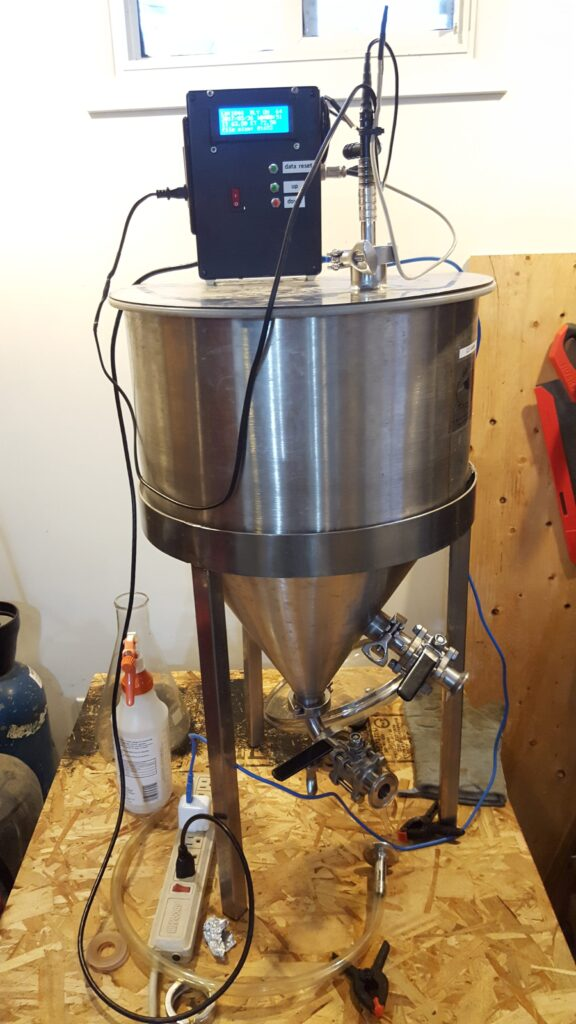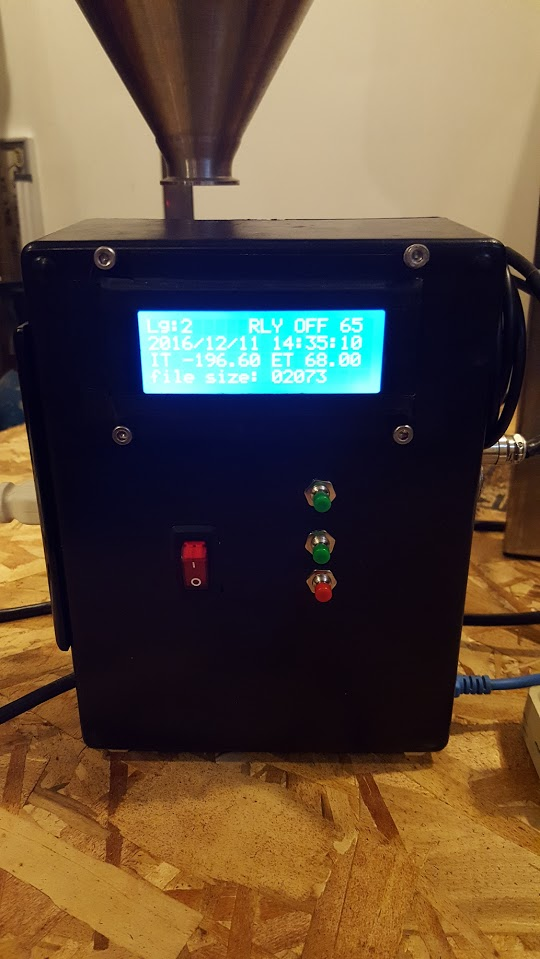# Beer + Arduino + # = #BeeruinoBeeruino – is an #arduino based data logger and controller project that I dreamt up after my second pint of #homebrew.  Here is an older video before the buttons were added for more flexible control.

What is it made of ?

• Black project box (Radio Shack), donated to me by a fellow brew head
• Originally using Arduino UNO R3 // Recently upgraded to the Mega 2560 R3 (more memory!)
• Ethernet Sheild (gives Ethernet and SD card capability)
• Prototyping shield
• RTC (real-time-clock) using I2C bus (give accurate date/time)
• dual 10 amp relay module (120 volt x 10 amp = 1200 watts)
• dual A/C sockets, independent of each other (so different things can be controlled out of each socket)
• 4×20 LCD screen using the I2C bus
• ON/OFF switch
• Arduino sketch C++ code
• USB Cable
• Power Cable to power the A/C sockets
• Analog buttons to change the set goal temperature UP or DOWN on the control relay, and to RESET the DATA storage on the SD card

Beeruino now has control buttons and Version 2 of the code has been released.  They allow you to control the target goal temperature without the need to change the value manually and no need to recompile the program using the computer, making it more independent and flexible as a tool.

All the versions of the code are maintained GitHub: https://github.com/rompstar/beeruino

Importing, Verifying, Analyzing and Plotting the Data:

I like to analyze the data using R (open-source) statistical software, that can do a lot more than just statistics, so don’t let that scare if you are not familiar or good with math/numbers.

Basically data in imported into R from the textfile.txt off the SD card, then I do some quick summary verification, I take a sample every 25 row and plot it in two different way using ggplot2.  Temperature of internal/external split by day and also the whole plot in one not segmented or split.

These two plot show a simple test run on plain water in a 12.5 gallon fermentor.

Started with cold 44F well water, turned on Beeruino and set temperature goal to 65F, then later raised it to 70F and again for the duration of the test to 75F.Here is a simple R script, this assumes you have some limited know-how in using R, if not do our self a favor and learn it.

```# import the data into R from the testfile.txt

class(BeerData) # should be data.frame
summary(BeerData)

# sample some data every 25th row from the whole data set
# so total_data / 25 are the number of observations used in the plots
BeerData2 = BeerData[seq(1, nrow(BeerData), 25), ]

# give columns more meaningful names
# load up the plyr package, install that if you are missing it
install.packages("plyr")
library(plyr)     # existing_name=new_name
BeerData3 <- rename(BeerData2, c("V1"="COUNTER", "V2"="HEAT_INDICATOR", "V3"="EXTERNAL_TEMP","V4"="INTERNAL_TEMP","V5"="DATE_TIME_STAMP"))

# Set up the Axis from the Variables
ext <- BeerData3\$EXTERNAL_TEMP # ext. temp.
int <- BeerData3\$INTERNAL_TEMP # int. temp.

summary(BeerData3)
install.packages("ggplot2")
library(ggplot2)

# plot to a file, (it won't diplay anything on the screen)
# if you want to display comment out the # png() and dev.off() rows...
png("whole_plot.png", width=480, height=480)

ggplot(BeerData3, aes(COUNTER, y=sensor_temperature, color = variable)) +
geom_line(aes(y = ext, col = "ext")) +
geom_line(aes(y = int, col = "int"))

dev.off()

png("day_split_plot.png", width=480, height=480)

ggplot(BeerData3, aes(COUNTER, y=sensor_temperature, color = variable)) +
geom_line(aes(y = ext, col = "ext")) +
geom_line(aes(y = int, col = "int")) +
facet_grid(as.Date(BeerData3\$DATE_TIME_STAMP, format="%Y/%m/%d") ~ .)

dev.off()
```

This entry was posted in Arduino-RaspberryPi-Make-Beer and tagged . Bookmark the permalink.

This site uses Akismet to reduce spam. Learn how your comment data is processed.